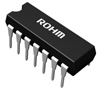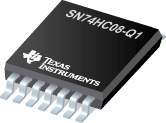Logic Gates InformationLogic gates are circuits with electronically controlled switches that combine digital signals according to Boolean algebra. In binary math, bits have only two possible values: 0 (off, false) and 1 (on, true). Consequently, there are three basic logic gates from which all other combinatorial logic functions are generated.

1. Inverters, or NOT gates, have only one input and reverse the logic state. A true input produces a false output, and vice versa.
2. AND gates perform a logical “and” operation on two inputs, A and B.
3. With OR gates, the variable Q equals 1 if A is 1, B is 1, or if both A and B are 1.

NOT, AND, and OR logic gates are considered to be functionally complete because they can be used to generate any function. Complex applications use numerous NOT, AND, and OR gates, along with NAND, NOR, XOR, and XNOR gates.

Logic Gates and Circuit Simplifcation Tutorial Video Credit: cslearning101

NAND logic gates serve as an AND gate followed by a NOT gate. In other words, NAND gates behave like the logical operator “and” followed by negation. The output is false if both inputs are true; otherwise, the output is true.

NOR gates behave like the logical operator "or" followed by negation. The output is true if both inputs are false; otherwise, the output is false.

XOR gates (exclusive-OR) behave like the logical operator “either / or”. The output is true if either, but not both, of the inputs are true. The output is false if both inputs are false, or if both inputs are true.

XNOR gates (exclusive-NOR) combine an XOR gate followed by an inverter. XNOR gates behave like the logical "either / or", operator followed by negation. The output is true if the inputs are the same, and false if the inputs are different.

Outputs

Logic gates provide several output methods. The output lines of 3-state logic gates can have three states: high, low, and high impedance.

• Complementary metal oxide semiconductor (CMOS) open drain outputs are counterparts to transistor-to-transistor logic (TTL) open collectors.
• Open drain devices require a pull-up resistor to achieve a true high state.
• Open collectors have an output signal provided by a transistor that acts like a switch closure to ground when activated.

Logic gates with complementary outputs have two outputs: the true output and its complement. For example, an OR gate with a complementary output produces both the true output and its complement.

Specifications

Logic gates vary in terms of supply voltage, logic family, and package type.

Supply voltage choices range from -5 V to 5 V, with many choices between these voltages.

Common logic families include standard, fast, high-speed and advanced CMOS; emitter coupled logic (ECL); TTL and Fairchild advanced Schottky TTL (FAST); gunning technology; and crossbar switch technology (CBT).

Common package types include ball grid array (BGA), quad flat package (QFP), single in-line package (SIP), and dual in-line package (DIP). Many packaging variants are available.In terms of features, some logic gates include buffered outputs or provide bus hold support that retains the bus’s last active status when the bus is disabled or does not have an active driver. Other logic gates are radiation-hardened or provide protection from electrostatic discharge (ESD). Devices with Schmitt triggers include circuitry that introduces hysteresis and counteracts noise.

Standards

JEDEC JEB 5 -- Methods of Measurement for Semiconductor Logic Gating Microcircuits

BS EN 60617-12 -- Graphical Symbols for Diagrams - Part 12: Binary Logic Elements

Image credits: